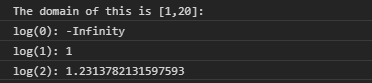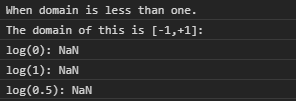# D3.js log.domain() Function

The log.domain() function is used to set the scale’s domain to the specified array of numbers. The array specified here must contain two or more than two elements. By default, the domain is [1,10].

Syntax:

`log.domain([domain]);`

Parameters: This function accepts single parameter as mentioned above and described below.

• [domain]: This is an array that takes two or more values that specifies the domain.

Return Values: This function does not return any value.

Below examples illustrate the log.domain() function in D3.js:

Example 1:

## HTML

 ` ` `<``html` `lang``=``"en"``> ` ` `  `<``head``> ` `    ``<``meta` `charset``=``"UTF-8"` `/> ` `    ``<``meta` `name``=``"viewport"` `path1tent``= ` `        ``"width=device-width, initial-scale=1.0"``/> ` `    ``<``script` `src``=``"https://d3js.org/d3.v4.min.js"``> ` `    `` ` ` ` ` `  `<``body``> ` `    ``<``script``> ` `        ``var log = d3.scaleLog() ` `         `  `            ``// Setting domain for the scale. ` `            ``// Domain can not be less than one. ` `            ``.domain([1, 20]) ` `            ``.range(["1", "2", "3", "4", "5"]); ` `        ``console.log("The domain of this is [1,20]: "); ` `        ``console.log("log(0): " + log(0)); ` `        ``console.log("log(1): " + log(1)); ` `        ``console.log("log(2): " + log(2)); ` `    `` ` ` ` ` `  ` `

Output:Example 2:

## HTML

 ` ` `<``html` `lang``=``"en"``> ` ` `  `<``head``> ` `    ``<``meta` `charset``=``"UTF-8"` `/> ` `    ``<``meta` `name``=``"viewport"` `path1tent``= ` `        ``"width=device-width,initial-scale=1.0"` `/> ` ` `  `    ``<``script` `src``=``"https://d3js.org/d3.v4.min.js"``> ` `    `` ` ` ` ` `  `<``body``> ` `    ``<``script``> ` `        ``var log = d3.scaleLog() ` `         `  `            ``// Setting domain for the scale. ` `            ``.domain([-1, +1]) ` `            ``.range(["1", "2", "3", "4", "5"]); ` `        ``console.log("When domain is less than one."); ` `        ``console.log("The domain of this is [-1,+1]: "); ` `        ``console.log("log(0): " + log(0)); ` `        ``console.log("log(1): " + log(1)); ` `        ``console.log("log(0.5): " + log(0.5)); ` `    `` ` ` ` ` `  ` `

Output:My Personal Notes arrow_drop_upCheck out this Author's contributed articles.

If you like GeeksforGeeks and would like to contribute, you can also write an article using contribute.geeksforgeeks.org or mail your article to contribute@geeksforgeeks.org. See your article appearing on the GeeksforGeeks main page and help other Geeks.

Please Improve this article if you find anything incorrect by clicking on the "Improve Article" button below.

Article Tags :

Be the First to upvote.

Please write to us at contribute@geeksforgeeks.org to report any issue with the above content.